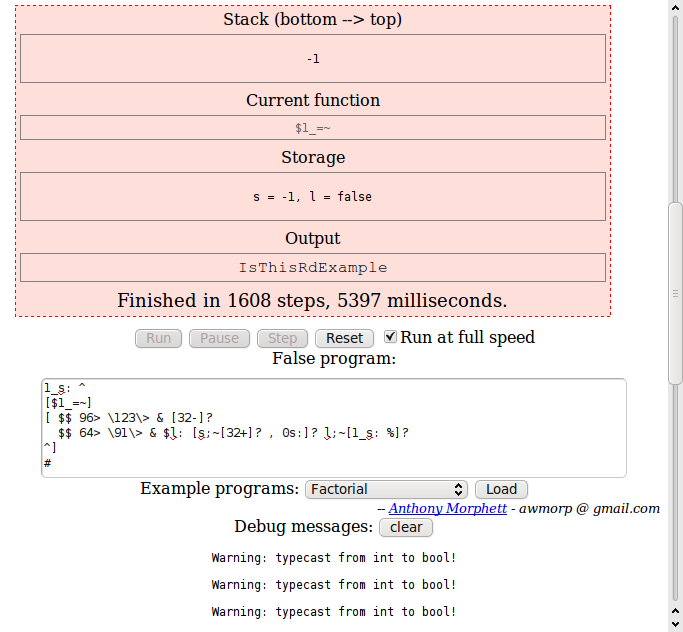# Morphett's FALSE

Implementation of programming language FALSE

An online interpreter created by Anthony Morphett. Is written in JavaScript. Differs from the original implementation in some details:

• allows multi-character variable names (only lowercase alphabet letters are allowed). However, to stick to the language spirit it is recommended to use long names only if all single-letter names are already used.
• a new `rotate` ® command is added which pops `N` from the stack and then moves `S<N>` to the top of the stack. This is done to fix the main inconvenience of the language — being unable to operate on stack elements except for top 3 ones.
• input/output are not buffered, so `flush` ß is not implemented.
• command-line arguments are not available.
• inline-assembly command ` is not implemented; instead this character can be used to set breakpoints for debugging.Morphett's FALSE interpreter (general appearance)

## Examples:

### Hello, World!:

Example for versions Morphett's FALSE, Wouter's FALSE 1.2.CF

A string is equivalent to a command which simply prints it. There’s no way to put the string on the stack, except for as a part of a function.

``````"Hello, World!"
``````

### Factorial:

Example for versions Morphett's FALSE, Wouter's FALSE 1.2.CF

This example uses iterative method of factorial calculation. Variables `i` and `f` store current number (loop counter) and current factorial value. After the variables are initialized, the loop starts. `[i;17=~]` defines the continuation condition: as long as `i` doesn’t equal 17. Loop body follows: `i` and a string constant are printed, `i` is incremented, old value of `f` is printed and new value of `f` is calculated.

For Wouter’s FALSE 1.2.CF factorial values for 13 and above are calculated with overflow error.

``````0i: 1f:
[i;17=~]
[i; \$."! = " 1+\$i: f;\$.10, *f:]
#
``````

### Fibonacci numbers:

Example for versions Morphett's FALSE, Wouter's FALSE 1.2.CF

This example uses iterative method of calculating Fibonacci numbers. Variables `a` and `b` store current numbers, variable `i` is loop counter.

The second loop empties the stack (deletes Fibonacci numbers written there by the first loop). Some interpreters tolerate data left in stack after program completion, but Wouter’s FALSE requires that the stack is empty and throws a runtime error otherwise.

``````0i: 1a: 1b:
[i;16=~]
[a; \$. ", " \$ b; \$ a: + b: i;1+i:]
#
"..."

[1=~]
[]
#
%
``````

### CamelCase:

Example for versions Morphett's FALSE

The input string is processed character by character. In Morphett’s FALSE strings are entered in a pop-up window, and end of input is marked by an empty line, which is then converted to `-1` value. That’s why `-1` is used for breaking the loop. Variable `s` stores “whether the previous character was a space” sign. Conditional commands execution (`?`) allows to run only `if-then` conditions; to implement `else` branch, one has to copy the condition (stored in variable `l` — whether the current character is a letter), invert it and use another `?`.

``````1_s: ^
[\$1_=~]
[ \$\$ 96> \123\> & [32-]?
\$\$ 64> \91\> & \$l: [s;~[32+]? , 0s:]? l;~[1_s: %]?
^]
#
``````